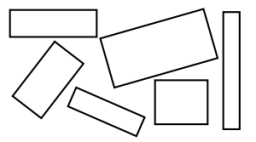# Rectangles 7346

Draw rectangles. Color them and calculate the circuits and areas

KLMN: KL = 5CM LM = 3CM

rectangle OPQR OP = 4cm PQ = 3.5cm

o1 =  16 cm
S1 =  15 cm2
o2 =  15 cm
S2 =  14 cm2

### Step-by-step explanation:Did you find an error or inaccuracy? Feel free to write us. Thank you!

#### Grade of the word problem:

We encourage you to watch this tutorial video on this math problem: